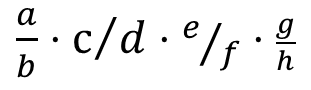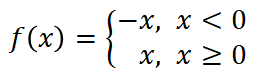Microsoft PowerPoint has an excellent equation tools for entering math equations. But if you have lots of equations to enter, it became tedious to go through the tool ribbon and switch back and forth between mouse click and keyboard.

It is certainly the case for me recently. So I decided figure out how to mainly use the keyboard to create these equations.

It turned out the Equation design tool already have something you can use to learn the basic sequences. As shown in the screenshot below, you can switch between different views of the equations.

• “Professional” view is what you normally see as the equation was input
• “Linear” view is the equation shown in a sequence of charactersFor example, the views for an matrix are shown belowHowever, we don’t have to directly input the special character (■). For the case of matrix, we can input as “\matrix”. So the sequence to input is below

here the & to separate elements, @ to separate rows. The special character will be created when space is typed in.

I created an incomplete table for different functions and how they should be input.

 Function Professional Linear Input Fractiona/b⋅c∕d⋅e⁄f⋅□(64&g/h) a/b\cdot … (only know the a/b part, c,d,e,f,g,h part still need to figure out how to) MatrixA=(■(1&2&3@4&5&6@7&8&9)) A=(\matrix(1&2&3@4&5&6@7&8&9)) Superscript/ Subscriptsa^b⋅c_d⋅e_g^f⋅(_i^h)j a^b\cdot c_d\cdot e_g^f\cdot (_i^h)j Radical√a+√(5&b) \sqrt a + \sqrt (5&b) Special Character αβγπΑΒΓΠ αβγπΑΒΓΠ \alpha \beta \gamma \pi \Alpha \Beta \Gamma \Pi Bracketsf(x)={█(-&x, x<0@&x, x≥0)┤ f(x)={\matrix(-&x, x<0@&x, x≥0)┤ (not sure how to input the last character ) Limitation and Loglim_(n→∞)⁡〖(1+1/n)^n 〗 lim_(n→∞)⁡〖(1+1/n)^n 〗

Although the table only cover a small portion of the equations, I hope it help you get some idea and then you can figure out how to use it for your advantage. You can always use the GUI first and then modify from the copied text.